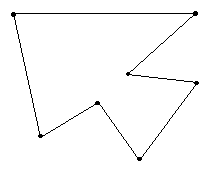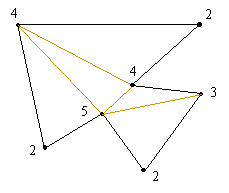## Project 1: Triangulated Polygons

Figure 1 shows a plane polygon with 7 sides.Figure 1

By adding diagonals, it becomes possible to triangulate the polygon, that is, to have all the regions after the diagonals are added consist of triangles (except for the unbounded region that surrounds the polygon, which will be a triangle only for the case when the original polygon has n = 3 sides).

One way of doing this for the polygon in Figure 1 is shown in Figure 2.Figure 2

One can now count the number of line segments that meet at each vertex of the polygon. The resulting numbers for the triangulated polygon in Figure 2 are shown in Figure 3.Figure 3

If the resulting collection of numbers is arranged in decreasing order one obtains what will be called the valence sequence of the triangulated polygon. The valence sequence for the triangulated polygon in Figure 3 is:

5, 4, 4, 3, 2, 2, 2.

Problem

1. Determine those integer sequences which can arise from some triangulated polygon.

Comment: Triangulated polygons are a special case of a class of graphs that can be drawn in the plane which are called outerplanar graphs. These are graphs for which all the vertices of the graph lie on a single face of the graph.

References:

1. Ore, O., Graphs and their Uses, Mathematical Association of America, Washington, 1963. (There is a new revised edition prepared by R. Wilson.)

2. West, D., Graph Theory, Prentice-Hall, Englewood Cliffs, 1996.

Joseph Malkevitch
Department of Mathematics and Computing
York College (CUNY)
Jamaica, New York 11451-0001
email: malkevitch@york.cuny.edu
(Comments and results related to the project above are welcome.)

Acknowledgements
Some of this work was prepared with partial support from the National Science Foundation (Grant Number: DUE 9555401) to the Long Island Consortium for Interconnected Learning (administered by SUNY at Stony Brook, Alan Tucker, Project Director).